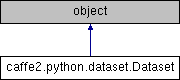Caffe2 - Python API A deep learning, cross platform ML framework
caffe2.python.dataset.Dataset Class Reference
Inheritance diagram for caffe2.python.dataset.Dataset:## Public Member Functions

def __init__ (self, fields, name=None)

def trim (self, net, multiple_of)

def init_empty (self, init_net)

def init_from_dataframe (self, net, dataframe)

def get_blobs (self)

def content (self)

def field_names (self)

def field_types (self)

def reader (self, init_net=None, cursor_name=None, batch_size=1, enforce_batch_size=False)

def random_reader (self, init_net=None, indices=None, cursor_name=None, batch_size=1, loop_over=False, enforce_batch_size=False)

def writer (self, init_net=None)

schema

fields

field_types

name

field_blobs

## Detailed Description

```Represents an in-memory dataset with fixed schema.

Use this to store and iterate through datasets with complex schema that
fit in memory.

Iterating through entries of this dataset is very fast since the dataset
is stored as a set of native Caffe2 tensors, thus no type conversion or
deserialization is necessary.
```

Definition at line 185 of file dataset.py.

## Constructor & Destructor Documentation

 def caffe2.python.dataset.Dataset.__init__ ( self, fields, name = `None` )
```Create an un-initialized dataset with schema provided by `fields`.

Before this dataset can be used, it must be initialized, either by
`init_empty` or `init_from_dataframe`.

Args:
fields: either a schema.Struct or a list of field names in a format
compatible with the one described in schema.py.
name: optional name to prepend to blobs that will store the data.
```

Definition at line 196 of file dataset.py.

## Member Function Documentation

 def caffe2.python.dataset.Dataset.content ( self )
```Return a Record of BlobReferences pointing to the full content of
this dataset.
```

Definition at line 256 of file dataset.py.

 def caffe2.python.dataset.Dataset.field_names ( self )
`Return the list of field names for this dataset.`

Definition at line 263 of file dataset.py.

 def caffe2.python.dataset.Dataset.field_types ( self )
```Return the list of field dtypes for this dataset.

If a list of strings, not a schema.Struct, was passed to the
constructor, this will return a list of dtype(np.void).
```

Definition at line 267 of file dataset.py.

 def caffe2.python.dataset.Dataset.get_blobs ( self )
```Return the list of BlobReference pointing to the blobs that contain
the data for this dataset.
```

Definition at line 248 of file dataset.py.

 def caffe2.python.dataset.Dataset.init_empty ( self, init_net )
```Initialize the blobs for this dataset with empty values.

Empty arrays will be immediately fed into the current workspace,
and `init_net` will take those blobs as external inputs.
```

Definition at line 228 of file dataset.py.

 def caffe2.python.dataset.Dataset.init_from_dataframe ( self, net, dataframe )
```Initialize the blobs for this dataset from a Pandas dataframe.

Each column of the dataframe will be immediately fed into the current
workspace, and the `net` will take this blobs as external inputs.
```

Definition at line 237 of file dataset.py.

 def caffe2.python.dataset.Dataset.random_reader ( self, init_net = `None`, indices = `None`, cursor_name = `None`, batch_size = `1`, loop_over = `False`, enforce_batch_size = `False` )
```Create a Reader object that is used to iterate through the dataset.

NOTE: The reader order depends on the order in indices.

Args:
init_net: net that will be run once to create the cursor.
cursor_name: optional name for the blob containing a pointer
to the cursor.
batch_size: how many samples to read per iteration.
loop_over: repeat the dataset indefinitely (in the same order)

Returns:
A DatasetReader that can be used to create operators that will
iterate through the dataset according to indices.
```

Definition at line 303 of file dataset.py.

 def caffe2.python.dataset.Dataset.reader ( self, init_net = `None`, cursor_name = `None`, batch_size = `1`, enforce_batch_size = `False` )
```Create a Reader object that is used to iterate through the dataset.

This will append operations to `init_net` that create a TreeCursor,
used to iterate through the data.

NOTE: Currently, it is not safe to append to a dataset while reading.

Args:
init_net: net that will be run once to create the cursor.
cursor_name: optional name for the blob containing a pointer
to the cursor.
batch_size: how many samples to read per iteration.

Returns:
A _DatasetReader that can be used to create operators that will
iterate through the dataset.
```

Definition at line 277 of file dataset.py.

 def caffe2.python.dataset.Dataset.trim ( self, net, multiple_of )
```Trims the contents of this dataset so that the number of records is
multiple of the given argument.
```

Definition at line 217 of file dataset.py.

 def caffe2.python.dataset.Dataset.writer ( self, init_net = `None` )
```Create a Writer that can be used to append entries into the dataset.

NOTE: Currently, it is not safe to append to a dataset